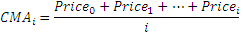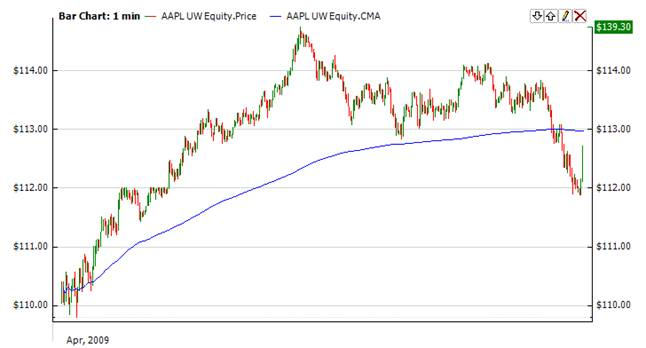﻿Cumulative Moving Average

# Cumulative Moving Average

The Cumulative Moving Average (Cma) is also frequently called a running average or a long running average although the term running average is also used as synonym for a moving average.

In some data acquisition systems, the data arrives in an orderly data stream and the statistician would like to calculate the average of all data up until the current data point, which in itself moves with time.  For example, an investor may want the average price of all trades for a particular financial instrument up until the current time.  As each new transaction occurs, the average price at the time of the transaction can be calculated for all transactions up to that point using the cumulative average.CalculationChart ExampleImplementation and Usage

Indicator has no input parameters. To initialize Cma use the following constructor:

Cma

Use

CMA - property to get current valueExample
C#
``` 1// Create new instance
2Cma cma = new Cma();
3
4// Number of stored values
5cma.HistoryCapacity = 2;
6